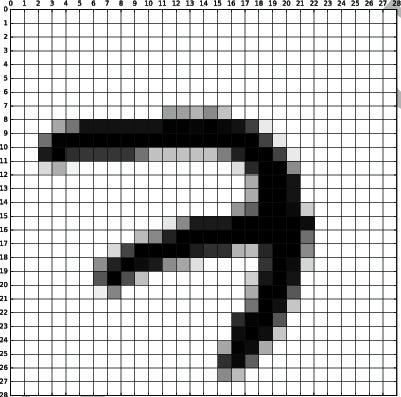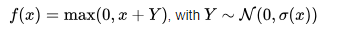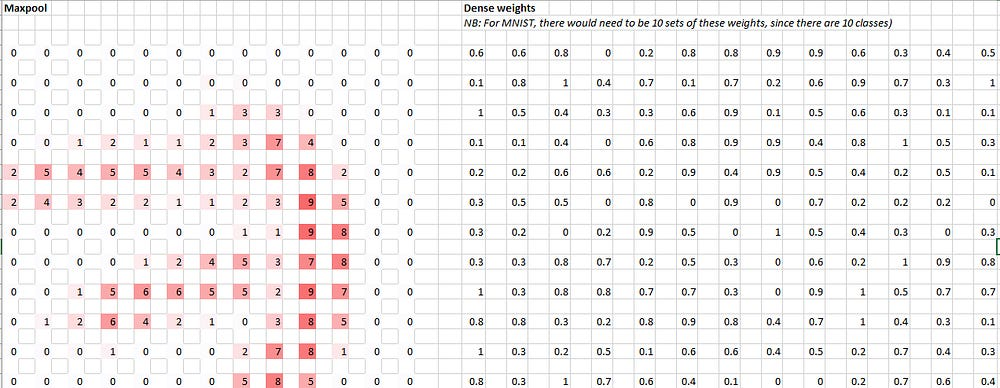# Convolutional Neural Networks In 10 Steps (lesson 3 fast.ai)

These are personal notes for the fast.ai deep learning part 1 course. These notes are a means for me to have some practice with the theory, and are written in an explanatory way.

Note: any sentence/word/phrase that ends with a ‘*’ is actually a misconception (“half-truth”) that is introduced to simplify the explanation. These misconceptions are then explained and corrected as we go on.

Get the spreadsheet from fast.ai here

### Convolutional Neural Network (CNN) Intro

Wikipedia defines convolutional neural networks as “feed-forward neural networks inspired by the connectivity patterns of the animal visual cortex.” As expected, it’s usually used for image classification problems. It’s actually the state of the art for these type of problems.

That sounds a lot cooler than how CNN’s are in practice. Let’s go over a CNN with an example from the MNIST dataset and an excel spreadsheet from fastai. For this example we’re going to be using the CNN architecture provided by the fastai library. Let’s say we have the following image of a number 7:

#### 1. Image to input

We take the “matrix representation” of that image and get a matrix of floats. For this example, each float represents a pixel. In excel, this matrix will look like the image above.

We will keep referring to this matrix as the input.

#### 2. First convolution

We also have what’s called a filter. In deep learning, a filter is often a 3×3 matrix* of weights:

Let’s call this filter a convolutional filter. This convolutional filter is then multiplied to every 3×3 piece of the input. This operation is called a convolutional operation. The filter above, when applied to the input, will look something like this:

This whole matrix is called a hidden layer. Each number in this matrix is called an activation. However, don’t get confused. Activations are numbers that must be computed using a convolutional filter.* This means that the numbers in the input are not activations.

This particular filter seems to be detecting the horizontal edges of a number 7. Here is another filter that detects more of the vertical edges:

It gives us the convolution

Let’s call these layers conv1 and conv2 respectively.

But where are the weights for the filters coming from?? These weights are learned using deep learning! There will be another post covering that.

#### 3. Clarifying activations: R.E.L.Uignore the Y for our purpose. x is the result of the convolutional operation as defined in 3

I actually held back on my definition of an activation. I said that “An activation is the result of a convolutional operation.” I left off the R.E.L.U part. R.E.L.U stands for Rectified Linear Unit. It’s a fancy term for the function above.

It’s just a function that is applied to the result of the convolutional operation such that if the result is < 0, just set the result to 0.

While its definition is simply that, we will get back to R.E.L.U later when we talk about non-linearities.

#### 4. Next step: another convolution

Now we have two convolutions or matrices composed of activations. We then take the next step and apply more filters to these convolutions. However, this time, each new convolution will be a linear combination whose terms are the results of the application operation of each 3×3 filter to both conv1 and conv2.

This is achieved by having 2 3×3 filters* with each filter responsible for calculating the activations from each convolution. Here, the top filter is for conv1 and the bottom filter is for conv2. Applying these two filters to the first hidden layer gives:

We also have two more filters for another layer. Let’s call these layers conv1’ and conv2’.

#### 5. Tensors

I actually held back on another defintion, this time on filters. Filters aren’t actually just 3×3 matrices, but instead are stored in tensors. Tensors are a mouthful to explain and this video does a nice job of doing that. For our purpose, tensors are just “stacks” of matrices. Imagine two coins on top of each other. For our purpose, each coin represents a 3×3 matrix. So, in step 4, the two separate 3×3 matrices are actually just parts of one 2x3x3 tensor — or a matrix whose components are made up of two 3×3 matrices.

#### 6. Maxpooling

In the CNN architecture we’re using we’re using another step called maxpooling. Maxpooling prevents overfitting. For our architecture, we’re using 2×2 maxpooling which means we take every 2×2 piece of a layer and take the highest activation of that piece. We’re left with a matrix which is half the resolution of the original matrix, but with similar activations.

#### 7. Fully connected layerleft: max pool layer, right: fully connected layer for the max pool layer on the left

Fully connected layers are matrices composed of weights for each activation in the max pool layer. Since we have two max pool layers, we will have two fully connected layers — one fully connected layer for each. We then take the sum product of each max pool layer/fully connected layer pair. We will then have two sum products: one for each max pool layer and fully connected layer. These sum products are then summed. This gives us a scalar dense activation.

Since we’re trying to classify the digit 7, and we have two max pool layers, we will have 10 pairs of dense weights — one pair for each digit (0–9). This means that we’ll get 10 different dense activations. In other words, we will repeat the process described above, 10 times, each time with a different pair of dense weights layer for each of the 10 digits (0–9) and end up with 10 scalars for each digit.

Try to visualize this process. It gets pretty hard to do this in excel.

#### 8. Getting probabilities. Part 1

In the end, all this CNN does is it calculates the probability that a digit is a 0,1,2…9 and then it guesses that it’s the digit with the highest probability. But how do we get that probability? We went from an image, to a matrix, and then more matrices, and now we have 10 seemingly random scalars. How can we get probabilities from that?

This is where all the steps above tie together. I like to think of the layers as “heat maps” that indicate which pixels were used or “activated” (hence the term “activation”). The fully connected layers are simply weights that were learned to check if the activations in the max pool layer resemble a 1,2,…9. Taking the sum product of an activation layer and a fully connected layer outputs a scalar dense activation which acts like an “arbitrary score” of how much the layer resembles a particular digit.

To turn this scalar “arbitrary score” to a probability, we apply another type of activation function called softmax.

#### The softmax activation function

This is the sigmoid activation function. As you can see, it’s simply a function that takes an arbitrary value and then “squashes” it between 0 and 1. Perfect for probabilities!!

The softmax function is a modified version of the sigmoid function which enables this “squashification” of arbitrary scalar values, but this time for K classes, and instead of squashing the values to the range [0,1], the range is now (0,1]. For our example above, we have K = 10 classes (the digits 0,1,…,9), we can take the 10 scalars we calculated, plug them into the softmax function and get probabilities for each class. How? Check this definition of softmax:

That looks scary 😨. However, it’s really quite simple. In simple terms, the top equation just says that if we have K classes, and thus will use the softmax function to calculate K values, the sum of those K values should add up to 1. Since softmax outputs will always add up to 1, it’s a great candidate for single label classification since it tends to choose a single z, and if there are ties, at most it will be 50/50.

The second part describes how we can actually calculate the probabilities. Simply put, we take a scalar, we take its exp(), repeat that for the remaining K-1 scalars, take their sum, and then divide the scalar of interest by that sum. Hmm, that doesn’t sound too clear. Let’s do an example.

#### 9. Getting probabilities part 2

Let’s say this time we’re predicting if an image is a cat, dog, plane, fish, or building. and we get the following output from our fully connected layer:

We then take the exp() of these dense activations and get

Notice how the exponential function got rid of negative values, and emphasized the differences between values. This behavior of the exponential function makes it very useful for generating probabilities for single label classification.

We then take the sum of the exp column, here that’s 8.45 and use it to calculate the softmax probabilities:

That’s definitely much less intimidating compared to the formal definition.

#### But what about multi-label classification?

Instead of softmax, we use the sigmoid function we introduced earlier and set a threshold of which probabilities will translate to picking a label.

#### 10. Why and non-linearities

Okay, now we have an idea of how convolutional neural networks work. But why do we do these steps? I feel like this video described neural networks in a simple and accurate way. Think of neural networks as children drawing lines on the sand to separate clusters of rocks. In our 7 example, we’re really just trying to create boundaries between datapoints. It just so happens that these datapoints belong in high-dimensional space, so we need a non-linear, high dimensional function to fit/separate these datapoints. This function is the neural network.

Above, we described convolutional neural networks as a pattern of linear operations (matrix operations) and applied non-linear functions (RELU, Softmax) to those linear results. I like to think that the linear combinations create “linear boundaries” while the non-linearities bend and curve those lines to better fit the data.

The video I linked actually explains this much better, and this website gives a visual proof.

#### Summary

We take the matrix representation of an image as input. We then create a first hidden layer by performing a convolutional operation with pre-trained filters and R.E.L.U. These hidden layers contain activations which act like heat maps that show which pixels are activated. After sufficient hidden layers, we perform a max-pooling, and then apply fully connected layers to calculate dense activations. Applying Softmax on these dense activations then gives us a probability for each image class.

Source: Deep Learning on Medium